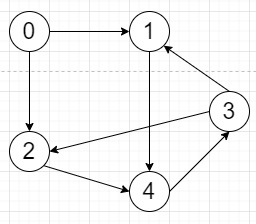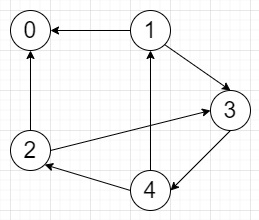# Program to reverse the directed graph in Python

Suppose we have a directed graph, we have to find its reverse so if an edge goes from u to v, it now goes from v to u. Here input will be an adjacency list, and if there are n nodes, the nodes will be (0, 1, ..., n-1).

So, if the input is likethen the output will beTo solve this, we will follow these steps −

• ans := a list of n different lists, where n is number of vertices
• for each index i, and adjacent list l in graph, do
• for each x in l, do
• insert i at the end of ans[x]
• return ans

Let us see the following implementation to get better understanding −

## Example

Live Demo

class Solution:
def solve(self, graph):
ans = [[] for _ in graph]
for i, l in enumerate(graph):
for x in l:
ans[x].append(i)
return ans
ob = Solution()
graph = [[1,2],,,[1,2],]
print(ob.solve(graph))

## Input

[[1,2],,,[1,2],]

## Output

[[], [0, 3], [0, 3], , [1, 2]]